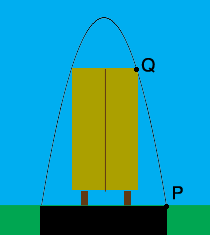SEARCH HOMEMath Central Quandaries & QueriesQuestion from Muhammad, a student: An arch over a road has a parabolic shape it is 6 meter wide at the base and is just tall enough to allow a truck 5 meter high and 4 meter wide to pass a): assuming that the arch has an equation of the form y=a(x)^2+b use the given information to find a & b. explain why this assumption is reasonable. b): sketch the graph of arch equationHi,

This is my diagram of the rear of the truck as it passes under the arch.The X-axis is along the surface of the ground and the Y-axis passes through the apex of the arch. What are the coordinates of the points $P$ and $Q?$ Substitute the coordinates of $P$ and $Q$ into $y = ax^2 + b$ and solve for $a$ and $b.$

PennyMath Central is supported by the University of Regina and the Imperial Oil Foundation.